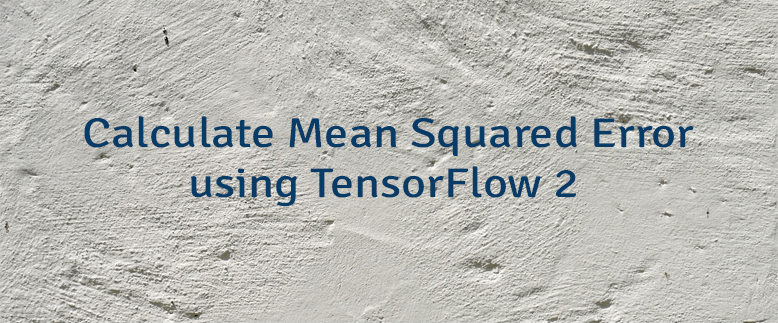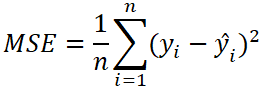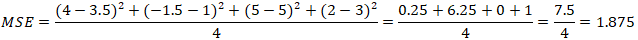# Calculate Mean Squared Error using TensorFlow 2

Mean squared error (MSE) is a loss function that is used to solve regression problems. MSE is calculated as the average of the squared differences between the actual and predicted values.

The formula to calculate the MSE:• `n` - the number of data points.
• `y` - the actual value of the data point. Also known as true value.
• `ŷ` - the predicted value of the data point. This value is returned by model.

Let's say we have the following sets of numbers:

Here is example how MSE can be calculated using these numbers:TensorFlow 2 allows to calculate the MSE. It can be done by using `MeanSquaredError` class.

``````from tensorflow import keras

yActual = [4, -1.5, 5, 2]
yPredicted = [3.5, 1, 5, 3]

mseObject = keras.losses.MeanSquaredError()
mseTensor = mseObject(yActual, yPredicted)
mse = mseTensor.numpy()

print(mse)``````

MSE also can be calculated by using `mean_squared_error`, `mse` or `MSE` function.

``````mseTensor = keras.losses.mean_squared_error(yActual, yPredicted)
mse = mseTensor.numpy()

mseTensor = keras.losses.mse(yActual, yPredicted)
mse = mseTensor.numpy()

mseTensor = keras.losses.MSE(yActual, yPredicted)
mse = mseTensor.numpy()``````